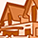cancel
Showing results for
Did you mean:Contributor

## Is there really a connection between the electromagnetic energy and the mechanical energy?

Dear all,

I would repeat the question that I posted in my previous blog "A missing link in atomic physics."

But before proceeding, please take a break and have some beverage, Sirs.

The question is this.

Please consider a tennis ball placed on your desk.  Assume that the ball has an electric charge q and an inertial mass m.  And think that you will grasp the ball by your hand and shake it repeatedly.

When shaking the ball that has a mass m, you need to apply accelerating force and decelerating force alternately.  That is busy and tiresome work.  You will feel a fatigue in your arm soon.  Thus, the work of shaking the ball must be consuming some mechanical energy.  The necessary energy may be calculable in some way.  This is the first point.

At the same time, when shaking the ball that has an electric charge q, you will be necessarily radiating electromagnetic energy toward the outer empty space.  This is the requirement from the modern electromagnetism.  As well as producing the electric field around the ball, the oscillating motion of the ball that has the electric charge q will generate the magnetic field too.  According to the modern electromagnetism, the vector product of the electric field and the magnetic field gives the Poynting vector which represents the electromagnetic energy flow in the empty vacuum space.  Imagine then, a big hollow sphere that surrounds you and may have a radius of 300,000km.  Due to the repeated oscillation of the ball, a net efflux of energy from such a sphere will occur.  That is, the surface integration of the Poynting vector on the surface of the sphere gives the energy efflux that is originated from the ball which you are shaking at the center of the sphere.  The total energy efflux which the shaken ball generates may also be calculable in some way.  Thi is the second point.

Now, it is the time for you to combine these two points and ponder about what the result will be.  I would like to ask you a question.  Are these two energies equal?  Is the loss of the mechanical energy that is consumed in shaking the ball really changed into the electromagnetic energy efflux?  If that were to be the case, then we must be able to derive a relation equation that equates the above two energy, one involving a factor q^2 and the other involving a factor m.  From such an equation, if we know the electric charge q, then we can purely theoretically calculate the inertial mass m, and vice versa.

Is this believable or unbelievable?  Or is there something wrong in the above arguments?  How do you think, Sirs?

Sincerely

3 RepliesNew Contributor III

## Re: Is there really a connection between the electromagnetic energy and the mechanical energy?

Mitsuru - Your statement of the problem is incorrect. It is missing some critical details. The two defining parameters of the "tennis ball" comprising the test object has mass m and charge q, but the values of these two quantities are independent of each other. Thus for any given mass m, the value of q can be anything from zero to some very large amount of charge. Since the mass of an electron is trivial compared to the mass of the tennis ball, adding charge will not affect the mass of the ball, although it will affect the strength of the electric field around the ball and therefore the strength of the EM energy radiated when the ball is oscillating. Therefore I find your statement that the mass of the ball can be determined from the strength of the field to be "unbelievable", since they have no relation to each other.Contributor

## Re: Is there really a connection between the electromagnetic energy and the mechanical energy?